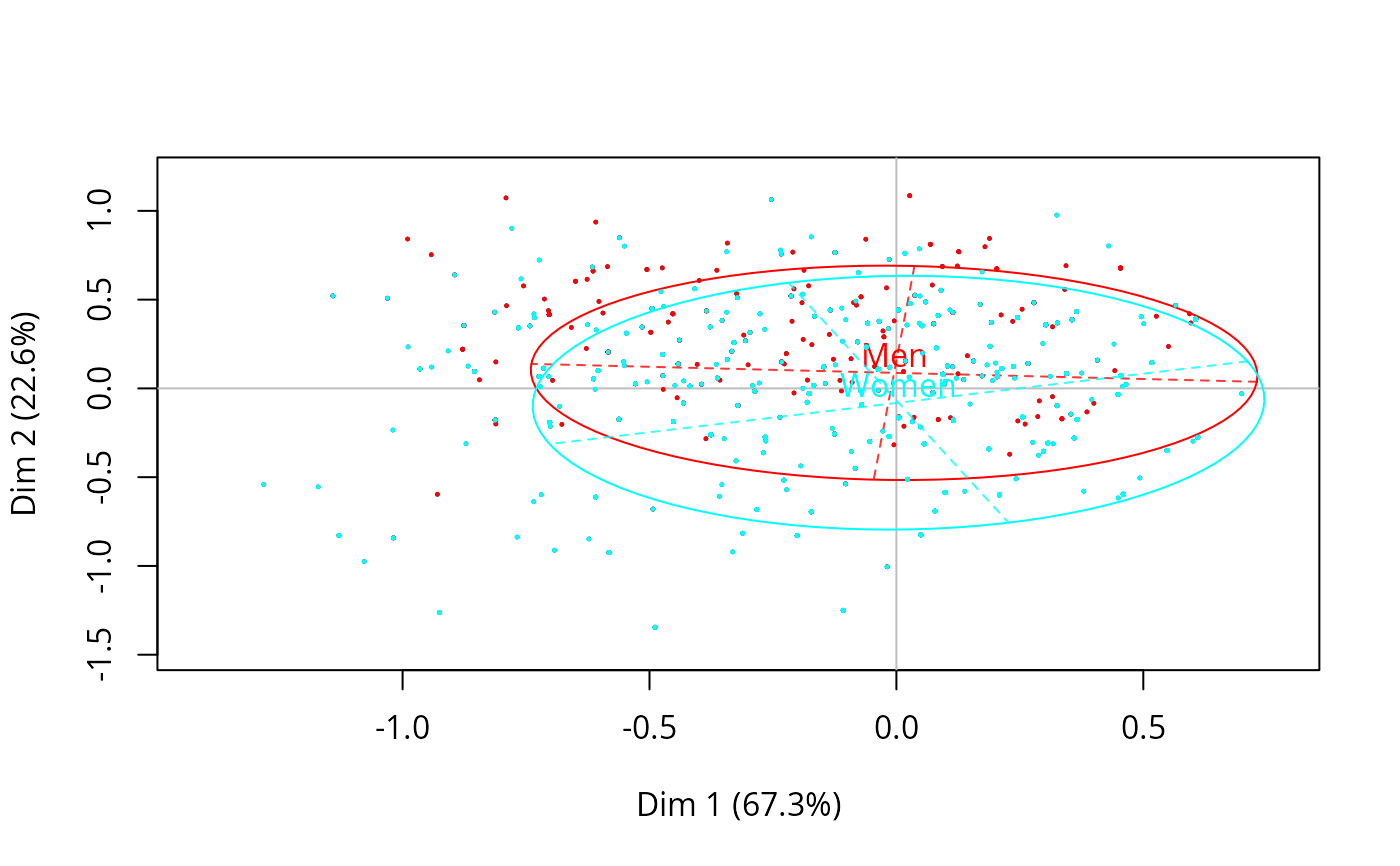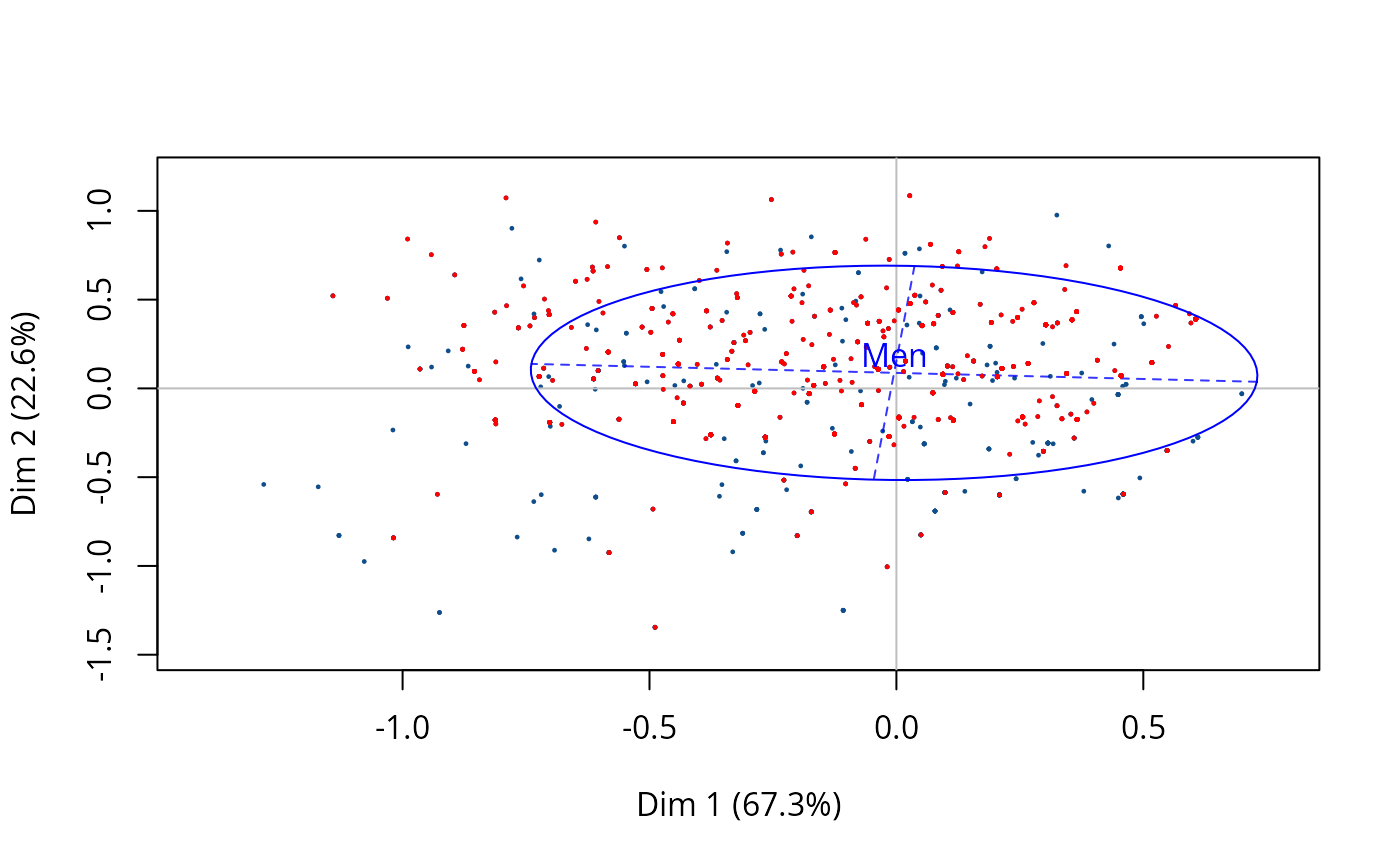Adds concentration ellipses or other kinds of inertia ellipses to the cloud of individuals of a MCA.

conc.ellipse(resmca, var, sel = 1:nlevels(var), axes = c(1, 2),
kappa = 2, col = rainbow(length(sel)), pcol = rainbow(length(sel)), pcex = 0.2,
lty = 1, lwd = 1, tcex = 1, text.lab = TRUE)

## Arguments

resmca

object of class MCA, speMCA, csMCA, multiMCA or stMCA

var

supplementary variable to plot

sel

numeric vector of indexes of the categories to plot (by default, ellipses are plotted for every categories)

axes

length 2 vector specifying the components to plot (default is c(1,2))

kappa

numeric. The kappa value (i.e. "index") of the inertia ellipses. By default, kappa = 2, which means that concentration ellipses are plotted.

col

vector of colors for the ellipses of plotted categories (by default, rainbow palette is used)

pcol

vector of colors for the points at the center of ellipses of plotted categories (by default, rainbow palette is used)

pcex

numerical value giving the amount by which points at the center of ellipses should be magnified (default is 0.2)

lty

line type for ellipses (default is 1)

lwd

line width for the ellipses (default is 1)

tcex

numerical value giving the amount by which labels at the center of ellipses should be magnified (default is 0.2)

text.lab

whether the labels at the center of ellipses should be displayed (default is TRUE)

## Details

If kappa=2, ellipses are called "concentration" ellipses and, for a normally shaped subcloud, contain 86.47 percents of the points of the subcloud. If kappa=1, ellipses are "indicator" ellipses and contain 39.35 percents of the points of the subcloud. If kappa=1.177, ellipses are "median" ellipses and contain 50 percents of the points of the subcloud. This function has to be used after the cloud of individuals has been drawn.

## Author

Nicolas Robette

plot.speMCA, plot.csMCA, plot.multiMCA, plot.stMCA

## Examples

## Performs specific MCA (excluding 'NA' categories) of 'Taste' example data set,
## plots the cloud of categories
## and adds concentration ellipses for gender variable
data(Taste)
junk <- c("FrenchPop.NA", "Rap.NA", "Rock.NA", "Jazz.NA", "Classical.NA",
"Comedy.NA", "Crime.NA", "Animation.NA", "SciFi.NA", "Love.NA",
"Musical.NA")
mca <- speMCA(Taste[,1:11], excl = junk)
plot(mca, type = "i")
conc.ellipse(mca, Taste$Gender)## Draws a blue concentration ellipse for men only plot(mca, type = "i") conc.ellipse(mca, Taste$Gender, sel = 1, col = "blue")